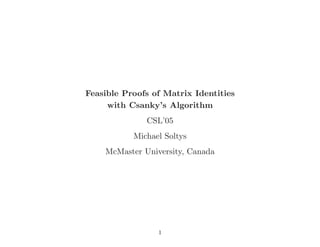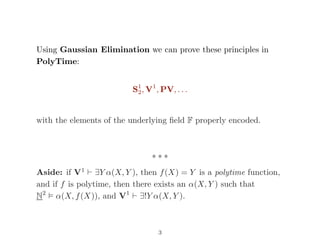Successfully reported this slideshow.

# Feasible proofs of matrix identities with csanky's algorithm - CSL 2005×

1 of 17
1 of 17

# Feasible proofs of matrix identities with csanky's algorithm - CSL 2005

## More Related Content

### Feasible proofs of matrix identities with csanky's algorithm - CSL 2005

1. 1. Feasible Proofs of Matrix Identities with Csanky’s Algorithm CSL’05 Michael Soltys McMaster University, Canada 1
2. 2. What is the complexity of the concepts needed to prove theorems of matrix algebra? For example: • AB = I ⊃ BA = I • The Cayley-Hamilton Theorem (If pA(x) = det(xI − A), then pA(A) = 0) • det(AB) = det(A) det(B) • Linear Independence (n + 1 vectors in Fn must be linearly dependent) 2
3. 3. Using Gaussian Elimination we can prove these principles in PolyTime: S1 2, V1 , PV, . . . with the elements of the underlying ﬁeld F properly encoded. ∗ ∗ ∗ Aside: if V1 ∃Y α(X, Y ), then f(X) = Y is a polytime function, and if f is polytime, then there exists an α(X, Y ) such that N2 α(X, f(X)), and V1 ∃!Y α(X, Y ). 3
4. 4. NC2 algorithms for computing the characteristic polynomial: 1. Berkowitz’s algorithm 2. “Newton’s Symmetric Polynomials” (Csanky’s) algorithm 3. Chistov’s algorithm Can we use them to give NC2 proofs of matrix properties? 4
5. 5. Berkowitz’s algorithm is based on Samuelson’s identity: A =        a R S M        p(x) = (x − a)q(x) − R · adj(xI − M) · S p and q are the char polys of A and M, respectively, and p = C1q 5
6. 6. For example, if A is a 4 × 4 matrix, then p = C1q is given by by:           p4 p3 p2 p1 p0           =           1 0 0 0 −a 1 0 0 −RS −a 1 0 −RMS −RS −a 1 −RM2 S −RMS −RS −a                  q3 q2 q1 q0        Berkowitz’s algorithm consists in repeating this for q, etc., and obtaining p as a product of matrices: p = C1C2 · · · Cn BerkA(x) = pnxn + pn−1xn−1 + · · · + p0. 6
7. 7. Csanky’s algorithm works as follows: s0 = 1, and for 1 ≤ k ≤ n, sk = 1 k k i=1 (−1)i−1 sk−itr(Ai ) So, s0 = 1 s1 = tr(A) s2 = 1 2 (s1tr(A) − s0tr(A2 )) s3 = 1 3 (s2tr(A) − s1tr(A2 ) + s0tr(A3 )) CsankyA(x) = s0xn − s1xn−1 + s2xn−2 − · · · ± snx0 . 7
8. 8. LA • Equality axioms x = x, x = y ⊃ y = x, . . . • Axioms for indices i + 1 = 0, i ∗ (j + 1) = (i ∗ j) + 1, . . . • Field axioms a + (−a) = 0, a ∗ b = b ∗ a, . . . • Axioms for – λij m, n, t operator for constructing matrices e.g., A + B := λij n, n, e(A, i, j) + e(A, i, j) – ΣA = sum of entries in A = a + ΣR + ΣS + ΣM • Rules for logical consequence, induction on open formulas (only bounded index quantiﬁcation), equality rules. 8
9. 9. LA proves ring properties of matrices (e.g., associativity of matrix multiplication), and can state, but apparently not prove: • AB = I ⊃ BA = I • [AB = I ∧ AC = I] ⊃ B = C • AB = I ⊃ [AC = 0 ⊃ C = 0] • AB = I ⊃ At Bt = I • If a column of A is zero, then for any B, AB = I However, LA can prove them equivalent. Open Problem: Show independence of any of the above from LA. 9
10. 10. LAP Add matrix powering function P to LA with the axioms P(0, A) = I and P(n + 1, A) = P(n, A) ∗ A Berk, Csanky can be expressed as terms of LAP. Open Problem: Can LAP prove the correctness of these algorithms? 10
11. 11. QLA Formulas with matrix quantiﬁers, and the axioms are all the theorems of LA. Shows the equivalences of: 1. The Cayley-Hamilton Theorem (CsankyA(A) = 0) 2. (∃B = 0)[AB = I ∨ AB = 0] 3. Linear Independence (n + 1 vectors in Fn must be linearly dependent) 4. Weak Linear Independence (nk vectors (n, k > 1) in Fn must be linearly dependent) 5. Every matrix has an annihilating polynomial 11
12. 12. Matrix powering can be expressed in QLA with POW(A, n): ∃B[B = B0 = I, B1, . . . , Bn ∧ (∀i ≤ n)(i < n ⊃ Bi+1 = Bi ∗ A)] And QLA (∃B = 0)[CB = I ∨ CB = 0] ⊃ POW(A, n) But QLA POW(A, n) Therefore QLA cannot prove (∃B = 0)[CB = I ∨ CB = 0], and so it cannot prove linear independence. 12
13. 13. QLA (∃B = 0)[CB = I ∨ CB = 0] ⊃ POW(A, n) Use the reduction of matrix powering to matrix inversea . Let N be an n2 × n2 matrix consisting of n × n blocks which are all zero except for n − 1 copies of A above the diagonal of zero blocks. Then, Nn = 0, and so (I − N)−1 = I + N + N2 + · · · + Nn−1 =         I A A2 . . . An−1 0 I A . . . An−2 ... I         aStephen Cook, “A taxonomy of problems with fast parallel algorithms,” Information and Computation, Vol. 64, 1985. 13
14. 14. Set C = I − N. Show that if CB = 0, then B = 0. (By induction on the rows of B, starting at the bottom.) Using (∃B = 0)[CB = I ∨ CB = 0] , conclude that there is a B such that CB = I. It remains to show that B = I + N + N2 + · · · + Nn−1 . (Again, by induction on the rows of B, starting at the bottom.) B contains I, A, A2 , . . . , An−1 in its top rows. POW(A, n) follows. 14
15. 15. On the other hand, QLA does not prove POW(A, n). Over the ﬁeld Z2, LA translates into AC0 . So, the “B” in POW(A, n) could be witnessed with an AC0  function, if QLA POW(A, n). But that would mean that DET(Z2) ⊆ AC0  — contradiction. (Because, “bit counting” is not in AC0 a , while it is in L ⊆ SL ⊆ DET(Z2)b .) aMod(3) is AC0 reducible to MAJORITY, and by the result of Razborov-Smolensky AC0  does not contain Mod(3), so it follows that MAJORITY is not in AC0 . bE. Gr¨adel, “Capturing Complexity Classes by Fragments of Second Order Logic,” Theoretical Computer Science, vol. 101, 1992. 15
16. 16. ∃LA Allows induction over formulas with bounded existential matrix quantiﬁers. P is deﬁnable in ∃LA ∃LA is a PolyTime theory ∃LA can prove all the principles stated thus far. Something better: let ∃PLA be the theory where induction is allowed only over formulas with bounded existential permutation quantiﬁers Then, ∃PLA still proves all these principles. 16
17. 17. Conclusion The Principle of Linear Independence is “all there is” to textbook Linear Algebra — proof complexity conﬁrms this conceptual intuition. LI cannot be shown trivially, i.e., in a simple theory such as QLA. A host of principles of Linear Algebra are QLA-equivalent to LI. LI can be shown with a PolyTime theory such as ∃LA. Open Question: Can LI be shown with an NC2 theory, for example LAP? 17